# fanimator

Create stop-motion animation object

Since R2019a

## Syntax

``fanimator(f)``
``fanimator(f,args)``
``fanimator(___,Name,Value)``
``fanimator(ax,___)``
``fp = fanimator(___)``

## Description

example

````fanimator(f)` creates a stop-motion animation object from the function `f`. The function `f` must return graphics objects that depend on only one variable. This variable defines the time parameter of the animation.By default, `fanimator` creates stop-motion frames of `f`(`t0`), generating 10 frames per unit interval of `t0` within the range of `t0` from 0 to 10.```

example

````fanimator(f,args)` allows the function `f` to depend on multiple variables. `args` specifies the input arguments of `f`.By default, the variable `t = sym('t')` is the time parameter of the animation. This syntax creates stop-motion frames of `f`(`subs`(`args`,`t`,`t0`)) within the range of `t0` from 0 to 10. You can animate a specific property of the graphics objects by setting its value to depend on `t` in the input argument `args`.```

example

````fanimator(___,Name,Value)` specifies the animation properties using one or more `Name,Value` pair arguments. Use this option with any of the input argument combinations in the previous syntaxes. Name-value pair settings apply to the animation object created.```

example

````fanimator(ax,___)` creates a stop-motion animation object in the axis specified by `ax` instead of in the current axis (`gca`). The option `ax` can precede any input argument combinations in the previous syntaxes.```
````fp = fanimator(___)` returns an `Animator` object. Use `fp` to query and modify the properties of a specific animation object. For a list of properties, see Animator Properties.```

## Examples

collapse all

Animate a point and a circle that move along a straight line.

First, create a function to plot a point at `(t,1)`. The variable `t` defines the time parameter of the animation.

```f = @(t) plot(t,1,'r*'); ```

Create a stop-motion animation object defined by `f`.

```fanimator(f) ```Next, create a function handle by using `fplot` to plot a unit circle. The circle is a function of two variables.

Create two symbolic variables `t` and `x`. Use `t` to set the center of the circle at `(t,1)` and `x` to parameterize the perimeter of the circle within the range `[-pi pi]`. Add the circle animation object to the existing plot. Set the x-axis and y-axis to be equal length.

```syms t x hold on fanimator(@fplot,cos(x)+t,sin(x)+1,[-pi pi]) axis equal hold off ```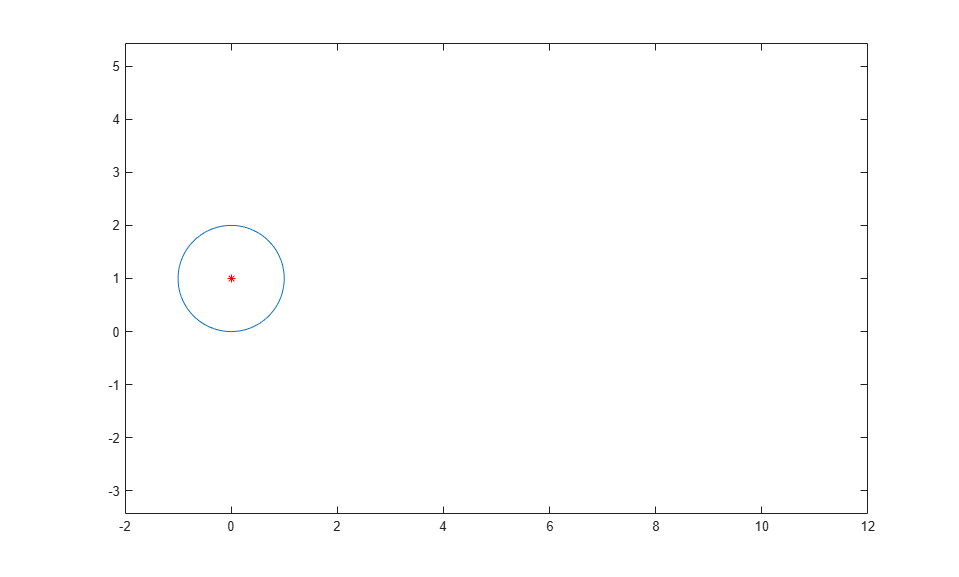Enter the command `playAnimation` to play the animation. By default, `fanimator` creates an animation object, generating 10 frames per unit time within the range of `t` from 0 to 10.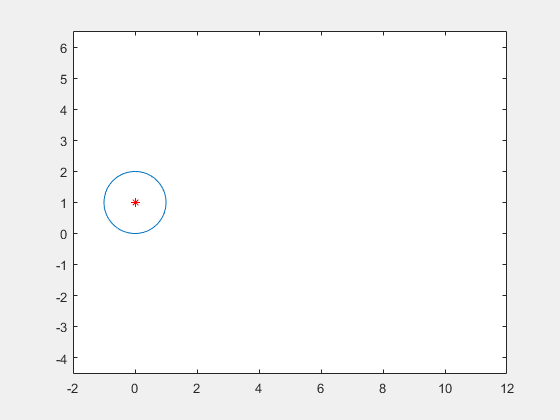Animate a line that changes vertical length and line width. You can animate a specific graphics property by setting its value to depend on the animation time parameter. By default, the variable `t` is the time parameter of the animation.

Create two symbolic variables, `y` and `t`. Plot a line with `y` coordinates within the interval `[0 t]` by using `fplot`. Use the `fanimator` function to create the line animation object. `fanimator` changes the line vertical length by increasing the value of `t` from 0 to 10.

```syms y t fanimator(@fplot,1,y,[0 t]) ```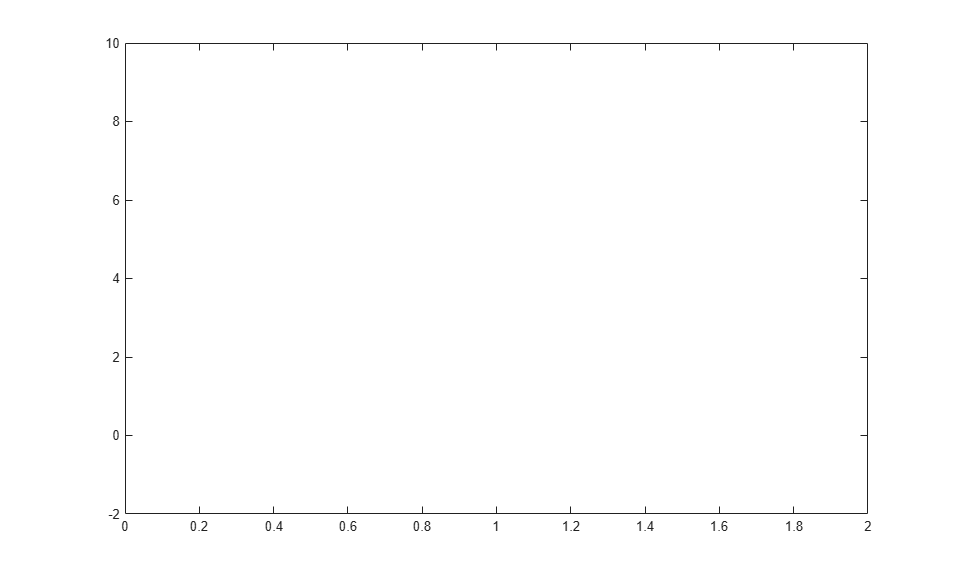Enter the command `playAnimation` to play the animation.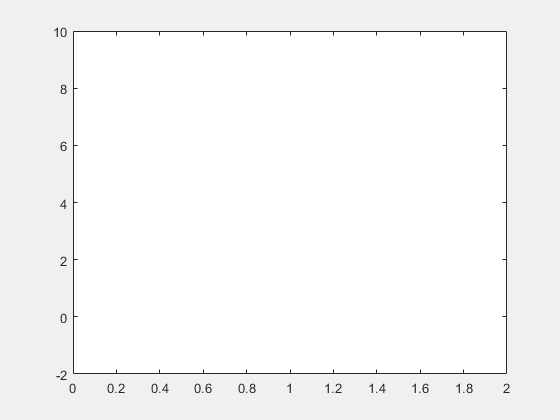Now plot a line with `y` coordinates within the interval `[0 2]` by using `fplot`. Set the `'LineWidth'` property value to `t+1`. Use the `fanimator` function to create the line animation object. `fanimator` changes the line width by increasing the value of `t` from 0 to 10.

```fanimator(@fplot,1,y,[0 2],'LineWidth',t+1) ```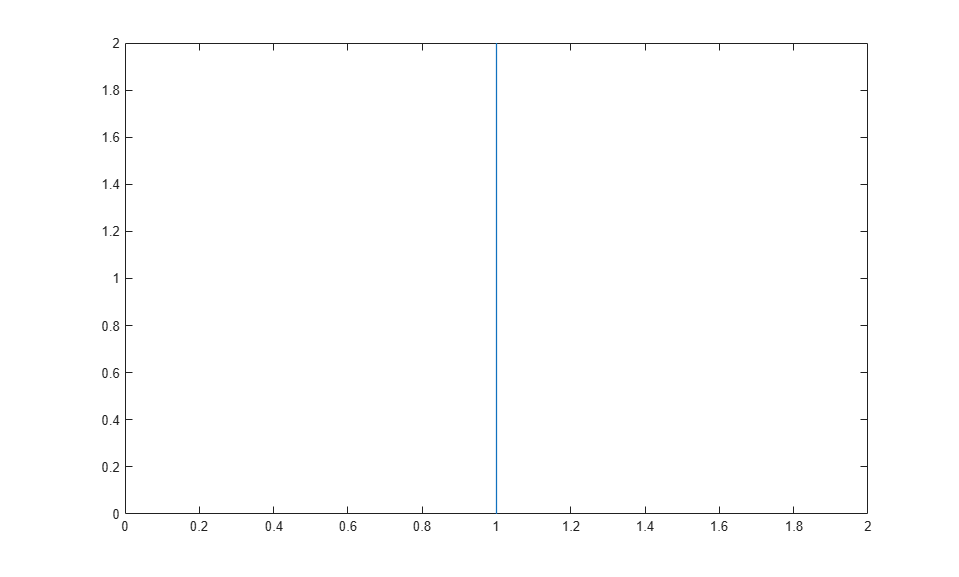Enter the command `playAnimation` to play the animation.Animate a circle with a timer.

First, create a function that plots a unit circle and save it in a file named `circ.m`. The function uses `fplot` to plot a unit circle centered at `(t,1)`, and the local symbolic variable `x` to parameterize the perimeter of the circle.

```function C = circ(t) x = sym('x'); C = fplot(cos(x)+t,sin(x)+1,[0 2*pi],'Color','red'); end ```

Use `fanimator` to create a unit circle animation object. Set the animation range of the time parameter to `[2 4.5]` and the frame rate per unit time to 4. Set the x-axis and y-axis to be equal length.

```fanimator(@circ,'AnimationRange',[2 4.5],'FrameRate',4) axis equal ```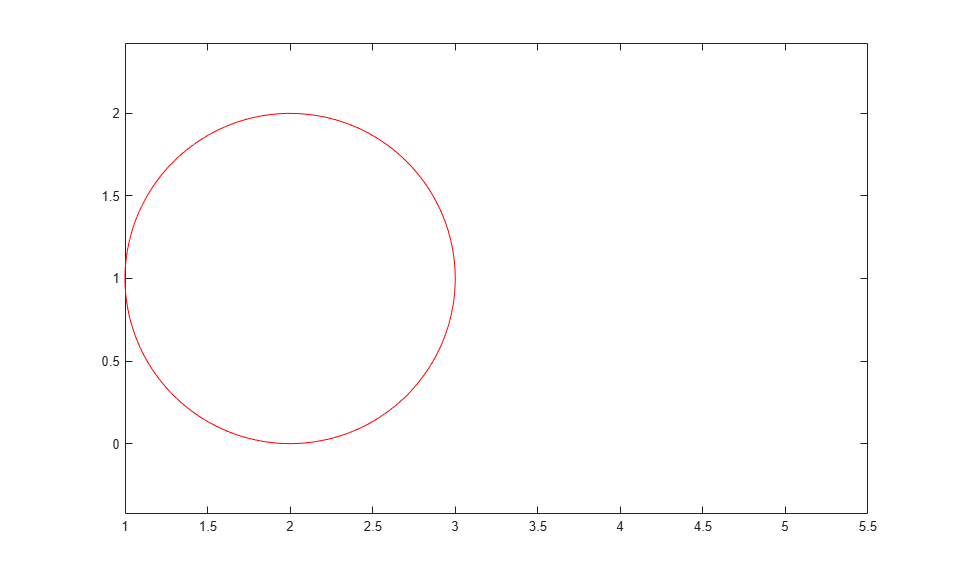Next, add a timer animation object. Create a piece of text to count the elapsed time by using the `text` function. Use `num2str` to convert the time parameter to a string. Set the animation range of the timer to `[0 4.5]`.

```hold on fanimator(@(t) text(4.5,2.5,"Timer: "+num2str(t,2)),'AnimationRange',[0 4.5]) hold off ```Enter the command `playAnimation` to play the animation. The timer counts the elapsed time from 0 to 4.5 seconds. The moving circle starts at 2 seconds and stops at 4.5 seconds.Animate two cycloids in separate axes. A cycloid is the curve traced by a fixed point on a circle as the circle moves along a straight line without slipping.

First, create two symbolic variables `x` and `t`. Create a figure with two subplots and return the first axes object as `ax1`. Create a moving circle animation object in `ax1` and add a fixed point on the rim of the circle. Set the x-axis and y-axis to be equal length.

```syms x t ax1 = subplot(2,1,1); fanimator(ax1, @fplot, cos(x)+t, sin(x)+1, [-pi pi]) axis equal hold on fanimator(ax1, @(t) plot(t-sin(t), 1-cos(t), 'r*')) ```To trace the cycloid, use a time variable in the plotting interval. The `fplot` function plots a curve within the interval `[0 t]`. Create the cycloid animation object. By default, `fanimator` creates stop-motion frames within the range of `t` from 0 to 10 seconds. `fanimator` plots the first frame at `t` equal to 0.

```fanimator(ax1, @fplot, x-sin(x), 1-cos(x), [0 t], 'k') hold off ```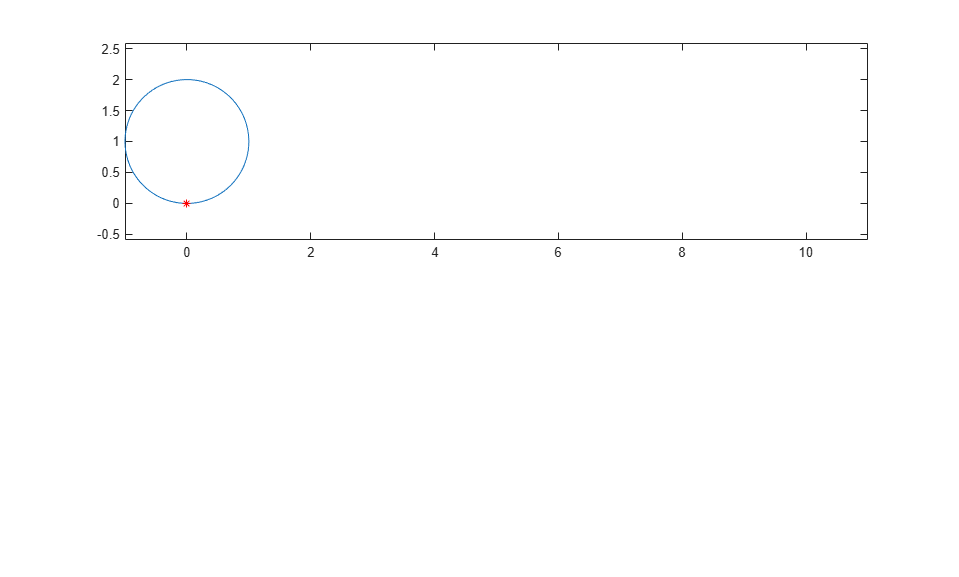Next, create another cycloid on the second axes object `ax2`. Trace the curve created by a fixed point at a distance of 1/2 from the center of the circle. Set the x-axis and y-axis to be equal length.

```ax2 = subplot(2,1,2); fanimator(ax2, @fplot, cos(x)+t, sin(x)+1, [-pi pi]) axis equal hold on fanimator(ax2, @(t) plot(t-sin(t)/2, 1-cos(t)/2, 'r*')) fanimator(ax2, @fplot, x-sin(x)/2, 1-cos(x)/2, [0 t], 'k') hold off ```Enter the command `playAnimation` to play the animation.Create a UI figure. Specify the UI axes of the figure.

```fig = uifigure; ax = uiaxes(fig);```Add an animation object to the UI axes using `fanimator`. Create two symbolic variables, `x` and `t`. Plot a curve that grows exponentially as a function of time `t` within the interval `[0 3]`.

```syms x t; fanimator(ax,@fplot,exp(x),[0 t],'r','AnimationRange',[0 3])```Play the animation in the UI figure `fig` by entering the command `playAnimation(fig)`. Alternatively, you can also use the command `playAnimation(ax.Parent)`.## Input Arguments

collapse all

Function returning graphics objects, specified as a function handle. For more information about function handles, see Create Function Handle.

Further arguments, specified as input arguments of a function handle that returns the graphics objects.

Target axes, specified as an `Axes` object. For more information about `Axes` objects, see `axes`.

### Name-Value Arguments

Specify optional pairs of arguments as `Name1=Value1,...,NameN=ValueN`, where `Name` is the argument name and `Value` is the corresponding value. Name-value arguments must appear after other arguments, but the order of the pairs does not matter.

Before R2021a, use commas to separate each name and value, and enclose `Name` in quotes.

Example: `'AnimationRange',[2 8],'FrameRate',30`

Animation time parameter, specified as a symbolic variable.

Example: `sym('y')`

Range of the animation time parameter, specified as a two-element row vector. The two elements must be real values that are increasing.

Example: `[-2 4.5]`

Frame rate, specified as a positive value. The frame rate defines the number of frames per unit time for the animation object.

Example: `30`

## Output Arguments

collapse all

Animation object, returned as a scalar. You can use this object to query and modify the properties of the generated animation frames. For a list of properties, see Animator Properties.

## Tips

• When you create a graph by using a plotting function, such as `fplot`, MATLAB® creates a series of graphics objects. You can then animate a specific property of the graphics objects by using the `fanimator` and the `playAnimation` functions. Note that some functions, such as `title` and `xlabel`, create text objects that cannot be animated. Instead, use the `text` function to create text objects that can be animated.

## Version History

Introduced in R2019a